Mechanical Engineering - Fluid Mechanics                                 Home : www.sharetechnote.com Fluid Mechanics : Bernoulli Equation   Bernoulli’s equation is a conservation of energy equation of a moving fluid per unit volume, which is equivalent to conservation of pressure for a moving fluid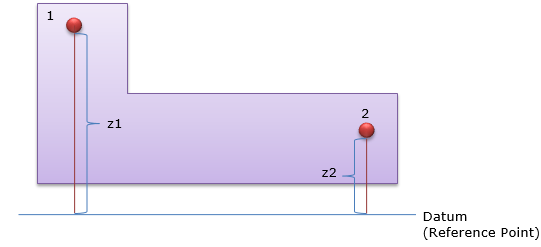The pressure equation of the point 1 and 2, and the relationship between the two points can be expressed as follows.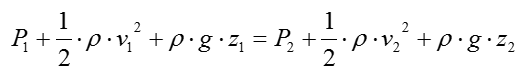Do you recall what each term in this equation indicates ? Short descrition on each term is labelled as below (For further details, refer to Pressure page)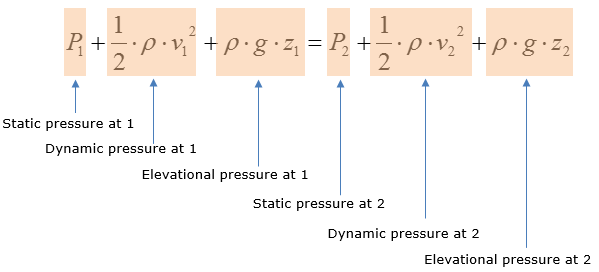The equation assumes the following: Steady-flow, fluid properties don’t change with time Incompressible flow, the density of fluid stays constant Friction due to viscous effects in the working fluid must be negligible No heat transfers with surroundings   Bernoulli Equation never used in control volume analysis due to the assumptions of no energy loss to heat, sound, incompressible fluid, etc Many problem solving questions with Bernoulli’s equation require the use of continuity law, which states that the volumetric flow rate (Q = Av) is constant Bernoulli Equation is often misused by engineers since they like to assume all the conditions that apply to the equation     Example 1 >   The bellmouth inlet shown in the drawing is used to measure flow rate into an air compressor. If the flow rate is 12,000 ft^3/min, what reading h will the U-Tube give in inches of water? Assume incompressible flow.   First recall that height difference of liquid in the U-Tube manometer is used to calculate the static pressure of the working fluid. In this case, the height difference in the manometer determines the static pressure of water at the inlet.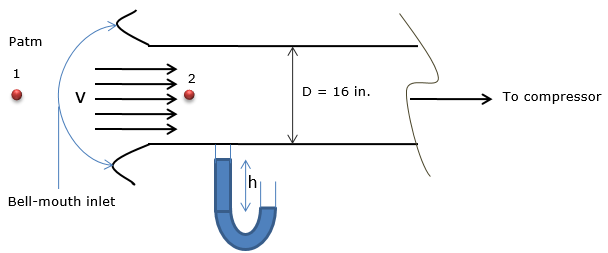Bernoulli’s equation is the only possible equation to calculate the static pressure at the bell-mouth inlet because there is no information on the losses in the working fluid and the fluid is assumed to be incompressible. Air is the working fluid in this case, as it flows into the bell-mouth inlet. Also assume 1D flow, where velocity of the working fluid does not vary in the vertical direction. Since you have to choose to work in inches or feet due to the givens, work in feet to calculate h then convert it back to inches. For fluid properties like density and gravitational acceleration, they are usually given in terms of feet, which makes calculations easier.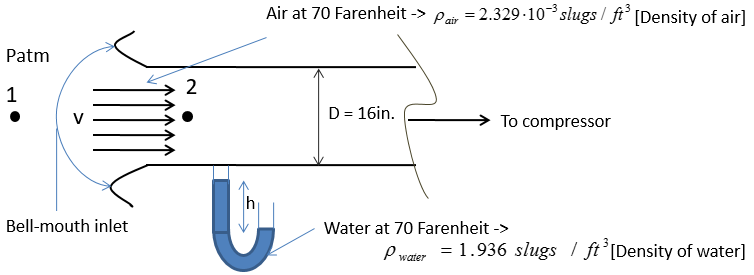As first step of the question, set the two points for Bernoulli analysis. Take point 1 at a long distance from the inlet and take point 2 at the inlet. Bernoulli’s equation of flow analysis is stated below, as the two points are now set for analysis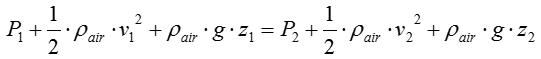At a long distance from the bell-mouth inlet, in which is close to the vacuum that the air is developed from, the velocity of air is negligible. -> v1 = 0. Ignore potential effects of air, meaning elevational changes are negligible-> z1 – z2 = 0. Then the equation is simplified to following due to the negligible air properties mentioned above: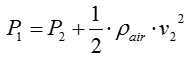Now that the equation is simplified, analyze the static pressure at point 1 and 2 as well as the dynamic pressure at point 2.   Consider the fluid properties at point 1: Since the air particles are far away from the bell-mouth inlet, the static pressure at that point is equal to the atmospheric pressure at 70 Farenheit is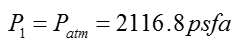Consider the fluid properties at point 2: Since the air particles are in the bell-mouth inlet at a relatively-high speed, the static pressure at that point is measured by the height difference of water in the U-Tube manometer. Since the height difference is what we are solving for, the expression for static pressure in absolute at point 2 corresponds to the hydrostatic pressure equation.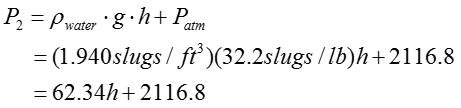The volumetric flow rate of air at the inlet is given as 12000 ft^3/min. Since volumetric flow rate is equal to cross-sectional area times velocity, the velocity at the inlet is equal to the volumetric flow rate over cross-sectional area, mathematically shown below: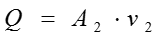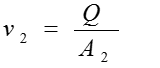Since diameter(D) of the inlet is given, the inlet has a circular cross-section for the air flow. Then the cross-sectional area in ft^2 is calculated as following: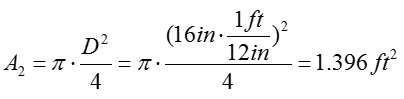Then,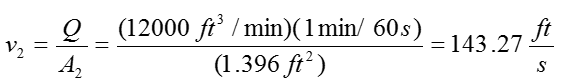Since all the fluid properties are known at this point, back-substitute all quantities into the simplified Bernoulli’s equation:Plugging in all the numbers you got in previous steps, you will get the following result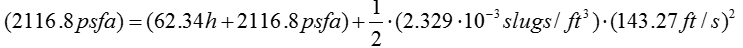You can cross out some numbers as shown below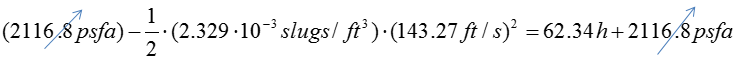You can simplify this even further as follows :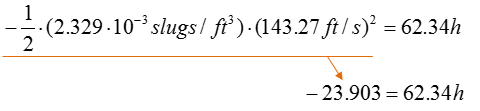You can rearrange this equation as follows. I take the absolute value since 'h' indicates the hight that cannot be negative.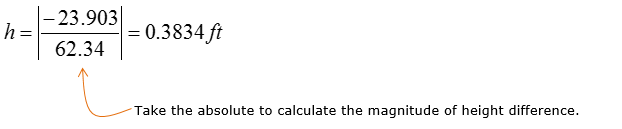Converting the unit from ft to in. you will get following result.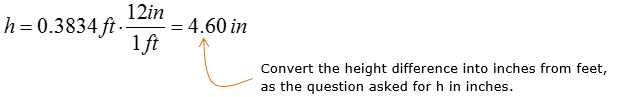Therefore the U-Tube manometer will give a reading of h = 4.60 in.